Welcome

It is highly recommended that you have Javascript enabled; many features will not work unless you do. The recommended browsers are Firefox and Chrome; the board is also NOT mobile-friendly.

If you were referred by someone (ex. me, Jessica) please put their username in the referral box on the registration page. Ask them if you don't know their username.

If you are visiting for TESTING PURPOSES ONLY, this is the test account information:

Test

Select a forum to post in:

## Unit Circle

Math topics

Moderators: Jessica, Teachers

Looking for a topic?
View the Math Topics Directory to find topics quicker under different kinds of math.

### Unit CircleThe 4 quadrants of a unit circle are just like your normal x,y coordinate planes...meaning the signs(+positive or -negative) for each (x ,y) value on a normal quadrant are the same as the signs for the (cos,sin) values on the unit circle.

In the first quadrant the x and y values, or in this case, sine and cosine values are both positive. The second quadrant has a negative cos and a positive sin. In the third one the cos and sin values are both negative. And in the last quadrant the cos value is positive and the sin value is negative.

This is how a normal 2π (360°) period breaks down.

Cosecant is the reciprocal of sine. 1/sine
Secant is the reciprocal of cosine. 1/cosine
Cotangent is the reciprocal of tangent. 1/tangent

Cosine = x so therefore cosecant would be 1/x. example: cos(2π) is 1. cosecant would be the reciprocal of that --> so csc(2π) is 1.

Sine = y so therefore secant would be 1/y. example: sin(2π) is 0. secant would be the reciprocal of that --> so secπ is undefined (0/0)

Tangent = y/x so therefore cotangent would be x/y. example: tan(π/4) is 1. cot(π/4) is 1.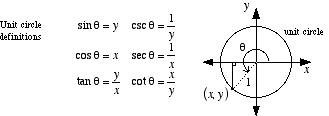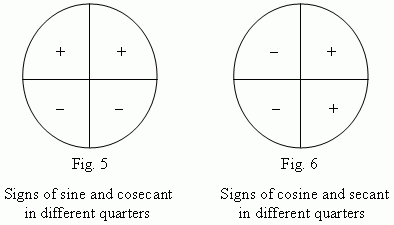• 0Jessica
Board Owner
Topic Author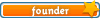Sapphire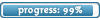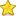Posts: 3,470
Topics: 1,244
Articles: 30
Joined: December 22nd, 2010, 8:04 pm